Exercises

# Fractions 2

Students will have a basic understanding of fractions coming into 4th grade. In this unit students will get to explore new ways of representing fractions, including in a set of data, on number lines and using area models. Students will use their knowledge of fractions to compare fractions with like and unlike denominators.
This lesson includes 5 printable learning activities.

## Fractions on a Number Line 2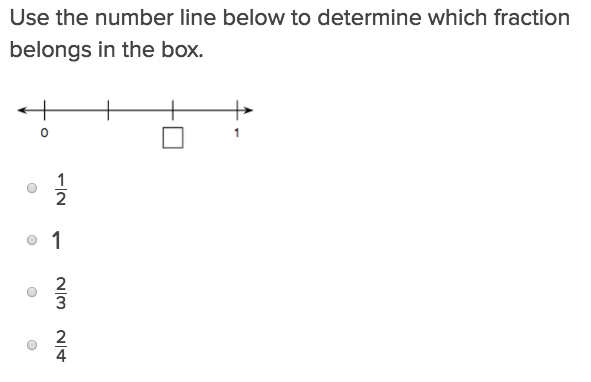Fractions on a Number Line 1 will help students practice this key third grade skill.

## Decimal Fractions 1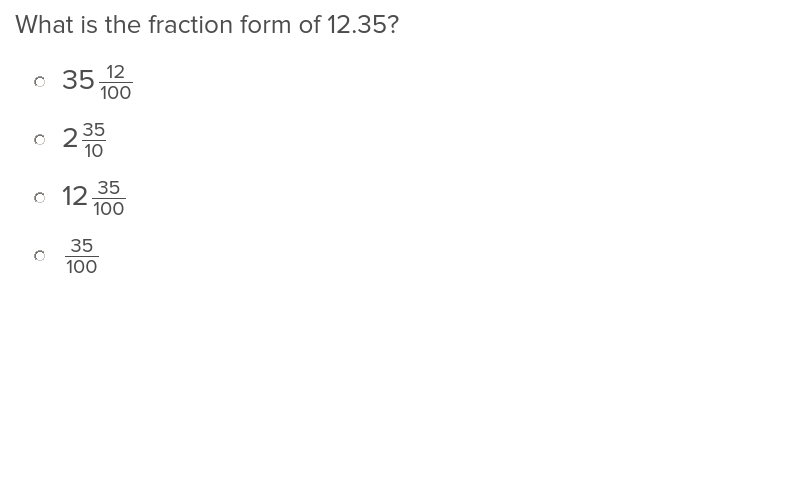Students will be able to convert from fractions to decimals, and back to fractions again with this exercise.

## Convert Decimals to Fractions 1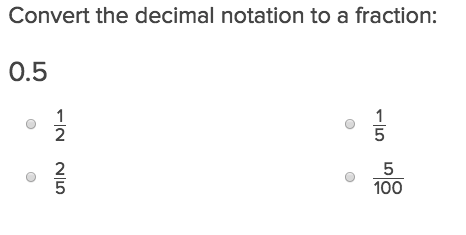Converting decimals to fractions will be a breeze for your students after they have completed this exercise.

## Fractions and Area Models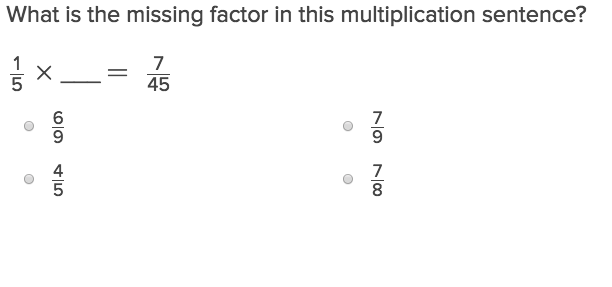Fractions and Area Models will help students practice this key fourth grade skill.

## Equivalent Fractions 2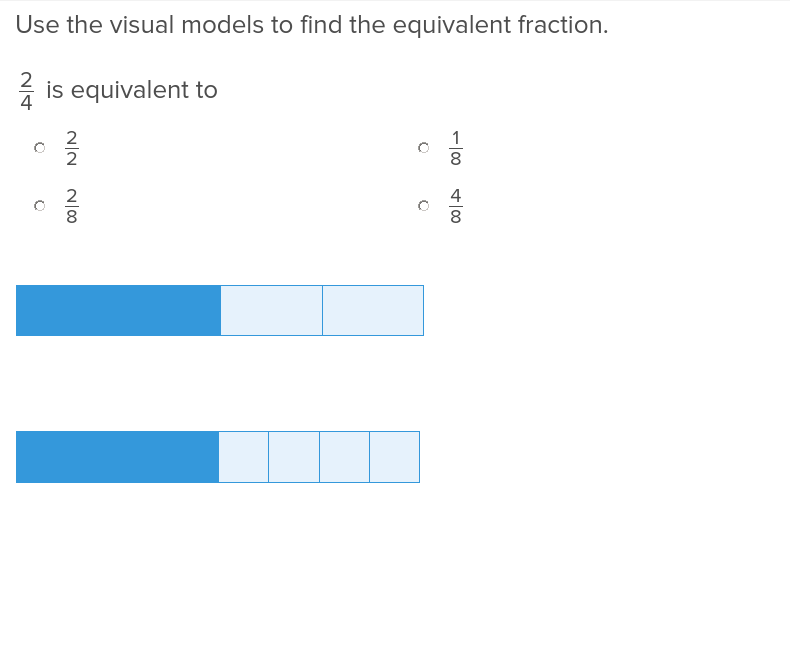Show students how to modify a math problem by finding equivalent fractions for any number.

## Comparing Fractions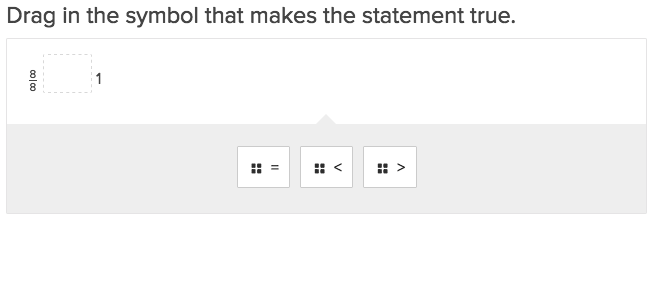Comparing Fractions will help students practice this key fourth grade skill.

## Galactic Space Fractions: Comparing Like Denominators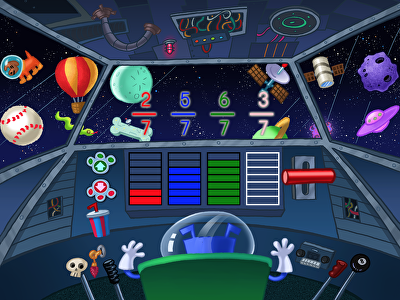In this galactic space fractions game, students compare like denominators to help Floyd get to planet Zorb. Students are asked to fill enough fuel cells to match the numbers represented in the fraction. Then they compare the fractions to determine which one is bigger or smaller. Comparing fractions is a key element of the math curriculum in fourth grade, in particular.

## Compare Fractions with the Same Denominators 2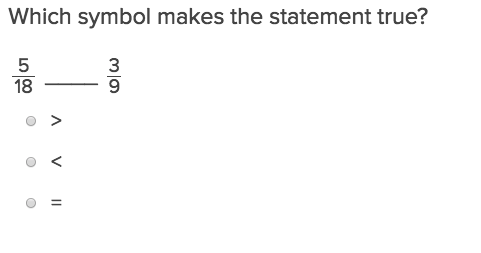All your students need to be able to compare fractions with the same denominators is this exercise.

## Compare Fractions with Different Denominators 2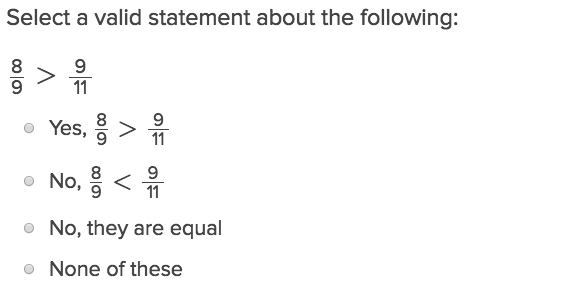Demystify comparing fractions with different denominators by giving your students access to this easy to understand exercise.

## Converting Fractions with Gems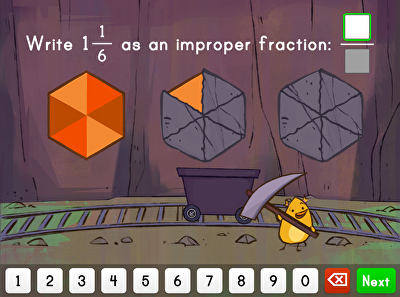In this game, students will practice converting fractions with gems. First, they must help Birdee uncover the correct fraction of each gem to match the mixed number shown. Then, students are asked to write the mixed number as an improper fraction, further deepening their comprehension of parts of a whole. Mastering mixed numbers and improper fractions is especially important in the fourth grade.

## Mixed Numbers and Improper Fractions 2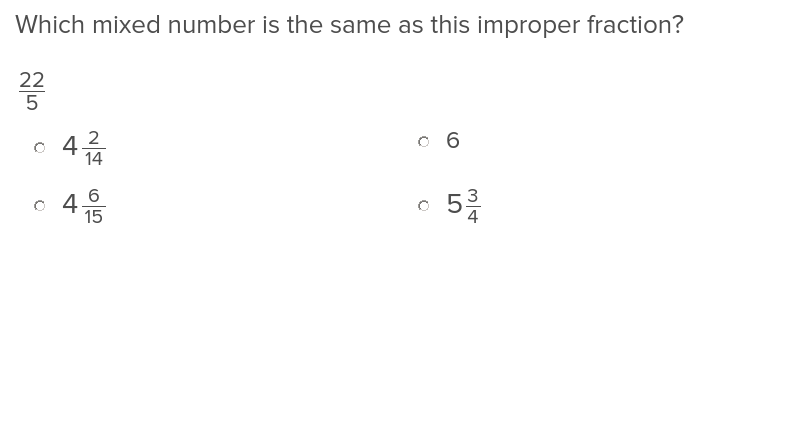Help students identify mixed numbers and improper fractions with this exercise that is easy to use and understand.

## Adding Fractions with Like Denominators: Jungle Edition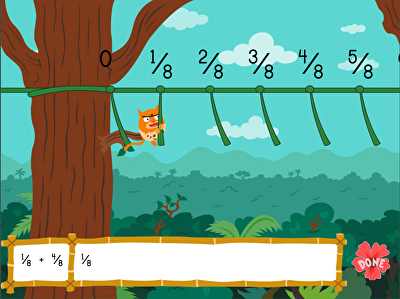Instead of swinging from the monkey bars, kids can swing through the jungle in this playful game that teaches adding fractions with like denominators. Correctly adding fractions will move Roly from vine to vine until he reaches the end. With funny sounds and engaging animations, this game makes a big fourth grade subject a little more interesting.

## Adding Fractions With the Same DenominatorThis exercise will show students how to add fractions properly by ensuring the denominators are like numbers.

## Subtracting Fractions with Like Denominators: City Edition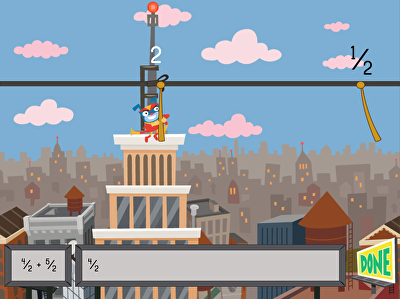Superhero Floyd needs to get to the next building to save the day! Help him get where he needs to go by subtracting fractions on a number line. Kids will become familiar with number lines and see how numbers line up next to each other. Floyd will save the day with some quick jumping and subtraction!

## Subtracting Fractions With Like DenominatorsTo properly subtract fractions, students will first need to work through this exercise demonstrating how to find like denominators.